## Multiplying Binomials

### Learning Outcomes

• Multiply binomials

Just like there are different ways to represent multiplication of numbers, there are several methods that can be used to multiply a binomial times a binomial.

### Using the Distributive Property

We will start by using the Distributive Property. Look again at the following example.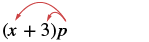We distributed the $p$ to get $x\color{red}{p}+3\color{red}{p}$ What if we have $\left(x+7\right)$ instead of $p$ ? Think of the $x+7$ as the $\color{red}{p}$ above.Distribute $\left(x+7\right)$ .Distribute again. ${x}^{2}+7x+3x+21$ Combine like terms. ${x}^{2}+10x+21$

Notice that before combining like terms, we had four terms. We multiplied the two terms of the first binomial by the two terms of the second binomial—four multiplications.

Be careful to distinguish between a sum and a product.

$\begin{array}{cccc}\hfill \mathbf{\text{Sum}}\hfill & & & \hfill \mathbf{\text{Product}}\hfill \\ \hfill x+x\hfill & & & \hfill x\cdot x\hfill \\ \hfill 2x\hfill & & & \hfill {x}^{2}\hfill \\ \hfill \text{combine like terms}\hfill & & & \hfill \text{add exponents of like bases}\hfill \end{array}$

### example

Multiply: $\left(x+6\right)\left(x+8\right)$

Solution

 $\left(x+6\right)\left(x+8\right)$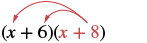Distribute $\left(x+8\right)$ . $x\color{red}{(x+8)}+6\color{red}{(x+8)}$ Distribute again. ${x}^{2}+8x+6x+48$ Simplify. ${x}^{2}+14x+48$

### try it

Now we’ll see how to multiply binomials where the variable has a coefficient.

### example

Multiply: $\left(2x+9\right)\left(3x+4\right)$

### try it

In the previous examples, the binomials were sums. When there are differences, we pay special attention to make sure the signs of the product are correct.

### example

Multiply: $\left(4y+3\right)\left(6y - 5\right)$

### try it

Up to this point, the product of two binomials has been a trinomial. This is not always the case.

### example

Multiply: $\left(x+2\right)\left(x-y\right)$

### Area Model for Multiplying Binomials

Now let’s explore multiplying two binomials. For those of you that use pictures to learn, you can draw an area model to help make sense of the process. You’ll use each binomial as one of the dimensions of a rectangle, and their product as the area.

The model below shows $\left(x+4\right)\left(x+2\right)$: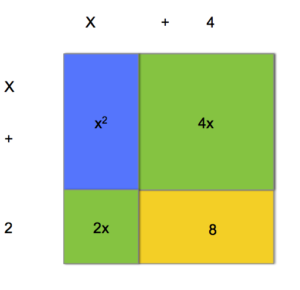Visual representation of multiplying two binomials.

Each binomial is expanded into variable terms and constants, $x+4$, along the top of the model and $x+2$ along the left side. The product of each pair of terms is a colored rectangle. The total area is the sum of all of these small rectangles, $x^{2}+2x+4x+8$, If you combine all the like terms, you can write the product, or area, as $x^{2}+6x+8$.

You can use the distributive property to determine the product of two binomials.

### Example

Simplify. $\left(x+4\right)\left(x+2\right)$

Look back at the model above to see where each piece of $x^{2}+2x+4x+8$ comes from. Can you see where you multiply $x$ by $x + 2$, and where you get $x^{2}$ from $x\left(x\right)$?

Another way to look at multiplying binomials is to see that each term in one binomial is multiplied by each term in the other binomial. Look at the example above: the $x$ in $x+4$ gets multiplied by both the $x$ and the $2$ from $x+2$, and the $4$ gets multiplied by both the $x$ and the $2$.

The following video provides an example of multiplying two binomials using an area model as well as repeated distribution.

## The Table Method

You may see a binomial multiplied by itself written as ${\left(x+3\right)}^{2}$ instead of $\left(x+3\right)\left(x+3\right)$.  To find this product, let’s use another method. We will place the terms of each binomial along the top row and first column of a table, like this:

 $x$ $+3$ $x$ $+3$

Now multiply the term in each column by the term in each row to get the terms of the resulting polynomial. Note how we keep the signs on the terms, even when they are positive, this will help us write the new polynomial.

 $x$ $+3$ $x$ $x\cdot{x}=x^2$ $3\cdot{x}=+3x$ $+3$ $x\cdot{3}=+3x$ $3\cdot{3}=+9$

Now we can write the terms of the polynomial from the entries in the table:

$\left(x+3\right)^{2}$

= $x^2$ + $3x$ + $3x$ + $9$

= $x^{2}$ + $6x$ + $9$.

Pretty cool, huh?

Polynomials can take many forms.  So far we have seen examples of binomials with variable terms on the left and constant terms on the right, such as this binomial $\left(2r-3\right)$.  Variables may also be on the right of the constant term, as in this binomial $\left(5+r\right)$.  In the next example, we will show that multiplying binomials in this form requires one extra step at the end.

### Example

Find the product.$\left(3–s\right)\left(1-s\right)$

## Using FOIL to Multiply BinomialsFoil Crane

Remember that when you multiply a binomial by a binomial you get four terms. Sometimes you can combine like terms to get a trinomial, but sometimes there are no like terms to combine. Let’s look at the last example again and pay particular attention to how we got the four terms.

$\left(x+2\right)\left(x-y\right)$
${x}^{2}-\mathit{\text{xy}}+2x - 2y$

Where did the first term, ${x}^{2}$, come from?

It is the product of $x\text{ and }x$, the first terms in $\left(x+2\right)\text{and}\left(x-y\right)$.The next term, $-\mathit{\text{xy}}$, is the product of $x\text{ and }-y$, the two outer terms.The third term, $+2x$, is the product of $2\text{ and }x$, the two inner terms.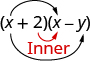And the last term, $-2y$, came from multiplying the two last terms.We can use a shortcut called the FOIL method when multiplying two binomials. Some people use the FOIL method to keep track of which pairs of terms have been multiplied when you are multiplying two binomials. This is not the same thing you use to wrap up leftovers, but an acronym for First, Outer, Inner, Last. It is called FOIL because we multiply the first terms, the outer terms, the inner terms, and then the last terms of each binomial.  The FOIL method arises out of using the distributive property to multiply two binomials. We are simply multiplying each term of the first binomial by each term of the second binomial and then combining like terms.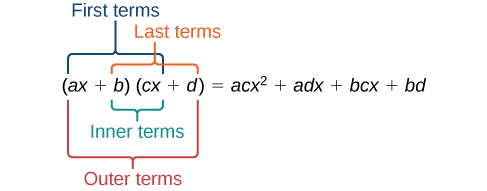Let’s go back to the example $\left(x+2\right)\left(x-y\right)$.  The following steps show you how to apply this method to multiplying two binomials.

$\begin{array}{l}\text{First}\text{ term in each binomial}: \,\,\,\,\,\,\,\,\,\,\,\,\,\,\left(x+2\right)\left(x-y\right)\,\,\,\,\,\,\,\,\,\,\,\,\,x\left(x\right)=x^{2}\\\text{Outer terms}:\,\,\,\,\,\,\,\,\,\,\,\,\,\,\,\,\,\,\,\,\,\,\,\,\,\,\,\,\,\,\,\,\,\,\,\,\,\,\,\,\,\,\,\,\,\,\,\,\,\,\,\,\,\left(x+2\right)\left(x-y\right)\,\,\,\,\,\,\,\,\,\,\,\,\,x\left(-y\right)=-xy\\\text{Inner terms}:\,\,\,\,\,\,\,\,\,\,\,\,\,\,\,\,\,\,\,\,\,\,\,\,\,\,\,\,\,\,\,\,\,\,\,\,\,\,\,\,\,\,\,\,\,\,\,\,\,\,\,\,\,\,\,\left(x+2\right)\left(x-y\right)\,\,\,\,\,\,\,\,\,\,\,\,\,2\left(x\right)=2x\\\text{Last terms in each binomial}:\,\,\,\,\,\,\,\,\,\,\,\,\,\left(x+2\right)\left(x-y\right)\,\,\,\,\,\,\,\,\,\,\,\,\,\,2\left(-y\right)=-2y\end{array}$

When you add the four results, you get the same answer, $x^{2}-xy+2x-2y$.

The last step in multiplying polynomials is to combine like terms.  In this example there were no like terms, but you will see this last step in several of the examples below. Remember that a polynomial is simplified only when there are no like terms remaining.Caution! Note that the FOIL method only works for multiplying two binomials together. It will not work for multiplying a binomial and a trinomial, or two trinomials.

### Example

Use FOIL to find the product. $(2x-18)(3x+3)$

The following steps summarize the process for using FOIL to multiply two binomials. It is very important to note that this process only works for the product of two binomials. If you are multiplying a binomial and a trinomial, it is better to use a table to keep track of your terms.

### Use the FOIL method for multiplying two binomials

1. Multiply the First terms.
2. Multiply the Outer terms.
3. Multiply the Inner terms.
4. Multiply the Last terms.
5. Combine like terms, when possible.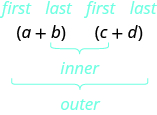### example

Multiply: $\left(y - 8\right)\left(y+6\right)$

### example

Multiply: $\left(2a+3\right)\left(3a - 1\right)$

### example

Multiply: $\left(5x-y\right)\left(2x - 7\right)$

### try it

In the following video, we show an example of how to use the FOIL method to multiply two binomials.

For another example of using the FOIL method to multiply two binomials watch the next video.

## Multiplying Two Binomials Using the Vertical Method

The FOIL method is usually the quickest method for multiplying two binomials, but it works only for binomials. You can use the Distributive Property to find the product of any two polynomials. Another method that works for all polynomials is the Vertical Method. It is very much like the method you use to multiply whole numbers. Look carefully at this example of multiplying two-digit numbers.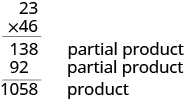You start by multiplying $23$ by $6$ to get $138$.

Then you multiply $23$ by $4$, lining up the partial product in the correct columns.

Last, you add the partial products.

Now we’ll apply this same method to multiply two binomials.

### example

Multiply using the vertical method: $\left(5x - 1\right)\left(2x - 7\right)$

### try itOrder Doesn’t Matter When You Multiply

One of the neat things about multiplication is that terms can be multiplied in either order. The expression $\left(x+2\right)\left(x+4\right)$ has the same product as $\left(x+4\right)\left(x+2\right)$, $x^{2}+6x+8$. (Work it out and see.) The order in which you multiply binomials does not matter. What matters is that you multiply each term in one binomial by each term in the other binomial.

Let’s look at another example using the FOIL method in which the variables have coefficients.

### Example

Simplify $\left(4x–10\right)\left(2x+3\right)$ using the FOIL acronym.

So far, we have shown several methods for multiplying two binomials together.  Why are we focusing so much on binomials?  They are one of the most well studied and widely used polynomials, so there is a lot of information out there about them. In the previous example, we saw the result of squaring a binomial that was a sum of two terms. In the next example we will find the product of squaring a binomial that is the difference of two terms.

### Example

Square the binomial difference $\left(x–7\right)$Caution! It is VERY important to remember the caution from the exponents section about squaring a binomial:

You can’t move the exponent into a grouped sum because of the order of operations!!!!!

INCORRECT: $\left(2+x\right)^{2}\neq2^{2}+x^{2}$

CORRECT: $\left(2+x\right)^{2}=\left(2+x\right)\left(2+x\right)$

In the video that follows, you will see another examples of using a table to multiply two binomials.

## Further Examples

The next couple of examples show you some different forms binomials can take.  In the first, we will square a binomial that has a coefficient in front of the variable, like the product in the first example on this page. In the second we will find the product of two binomials that have the variable on the right instead of the left. We will use both the FOIL method and the table method to simplify.

### Example

Find the product. $\left(2x+6\right)^{2}$

In the last example, we want to show you another common form a binomial can take, each of the terms in the two binomials is the same, but the signs are different. You will see that in this case, the middle term will disappear.

### Example

Multiply the binomials. $\left(x+8\right)\left(x–8\right)$

There are predictable outcomes when you square a binomial sum or difference. In general terms, for a binomial difference,

$\left(a-b\right)^{2}=\left(a-b\right)\left(a-b\right)$,

the resulting product, after being simplified, will look like this:

$a^2-2ab+b^2$.

The product of a binomial sum will have the following predictable outcome:

$\left(a+b\right)^{2}=\left(a+b\right)\left(a+b\right)=a^2+2ab+b^2$.

The product of a binomial sum and binomial difference of the same two monomial will have the following predictable outcome:

$\left(a+b\right)\left(a-b\right)=a^2-b^2$.

Note that a and b in these generalizations could be integers, fractions, or variables with any kind of constant.  You will learn more about predictable patterns from products of binomials in later math classes.

In this section we showed how to multiply two binomials using the distributive property, an area model, by using a table, using the FOIL method, and the vertical method.  Practice each method, and try to decide which one you prefer.

Some of the forms a product of two binomials can take are listed here:

• $\left(x+5\right)\left(2x-3\right)$
• $\left(x+7\right)^{2}$
• $\left(x-1\right)^{2}$
• $\left(2-y\right)\left(5+y\right)$
• $\left(x+9\right)\left(x-9\right)$
• $\left(2x-4\right)\left(x+3\right)$

And this is just a small list, the possible combinations are endless. For each of the products in the list, using one of the two methods presented here will work to simplify.

## Summary

Multiplication of binomials and polynomials requires an understanding of the distributive property, rules for exponents, and a keen eye for collecting like terms. Whether the polynomials are monomials, binomials, or trinomials, carefully multiply each term in one polynomial by each term in the other polynomial. Be careful to watch the addition and subtraction signs and negative coefficients. A product is written in simplified form if all of its like terms have been combined.

## Contribute!

Did you have an idea for improving this content? We’d love your input.